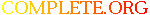Complete.Org: Mailing Lists: Archives: freeciv-ai: November 2002: [freeciv-ai] Re: Measure the AI ability to harvest resources# [freeciv-ai] Re: Measure the AI ability to harvest resources

[Top] [All Lists]

 To: Gregory Berkolaiko Cc: freeciv-ai@xxxxxxxxxxx Subject: [freeciv-ai] Re: Measure the AI ability to harvest resources From: Raimar Falke Date: Mon, 25 Nov 2002 15:10:11 +0100

```On Mon, Nov 25, 2002 at 12:47:46PM +0000, Gregory Berkolaiko wrote:
> On Sun, 24 Nov 2002, Raimar Falke wrote:
>
> > all 4 player are controlled by server-AI:
> >
> > 2: 29:            Wang Gon:(f s t)=(  75  125  138) (l t s)=(   0   11
> > 127) s=  5206 uf= 429
> > 2: 29:      Guntis Ulmanis:(f s t)=( 113  147  146) (l t s)=(   0    1
> > 145) s=  6398 uf= 440
> > 2: 29:   Richard Lionheart:(f s t)=( 112  189   59) (l t s)=(   0   25
> > 34) s=  6049 uf= 499
> > 2: 29:             Genghis:(f s t)=( 105   60  144) (l t s)=(   0    0
> > 144) s=  4743 uf= 408
> >
> > Agents (CMA, SMA and EA) control Genghis (the player with the lowest
> > rating):
> > 2: 29:            Wang Gon:(f s t)=(  75  125  138) (l t s)=(   0   11
> > 127) s=  5206 uf= 441
> > 2: 29:      Guntis Ulmanis:(f s t)=( 113  147  146) (l t s)=(   0    1
> > 145) s=  6398 uf= 443
> > 2: 29:   Richard Lionheart:(f s t)=( 112  189   59) (l t s)=(   0   25
> > 34) s=  6049 uf= 493
> > 2: 29:             Genghis:(f s t)=(  87  187   90) (l t s)=(   0   13
> > 77) s=  5912 uf= 438
> > +25%
>
> Threefold increase in production but twofold slump in science!  Can CMA be
> adjusted so that both science and production are increased?

It can. But I don't think that this is a good idea. We are here
speaking of the first 30 turns. IMHO it is better to produce settlers
here to found cities. I have no facts to back this up.

> > agents control Guntis (highest rating):
> > 2: 29:            Wang Gon:(f s t)=(  75  125  138) (l t s)=(   0   11
> > 127) s=  5206 uf= 445
> > 2: 29:      Guntis Ulmanis:(f s t)=( 125  223  105) (l t s)=(   0   28
> > 77) s=  7426 uf= 423
> > 2: 29:   Richard Lionheart:(f s t)=( 112  189   59) (l t s)=(   0   25
> > 34) s=  6049 uf= 493
> > 2: 29:             Genghis:(f s t)=( 105   60  144) (l t s)=(   0    0
> > 144) s=  4743 uf= 413
> > +16%
>
> Same here, huge slump in science, and an increase of production.
>
> > To have a fairer ground for comparing (and also to see how bad/good my
> > EA is) I now show all the players the map at the start of the game.
>
> What does that mean???

show_map_to_all is called before the game starts.

> > 2: 29:            Wang Gon:(f s t)=(  71  147  150) (l t s)=(   0   42
> > 108) s=  5648 uf=4000
> > 2: 29:      Guntis Ulmanis:(f s t)=( 123  160  162) (l t s)=(   0   11
> > 151) s=  7001 uf=4000
> > 2: 29:   Richard Lionheart:(f s t)=( 108  195   92) (l t s)=(   0   30
> > 62) s=  6471 uf=4000
> > 2: 29:             Genghis:(f s t)=( 145  173  139) (l t s)=(   0   19
> > 120) s=  7364 uf=4000
> >
> > All AIs perform better. Genghis is +55%. I thought that the AI is
> > omniscience.
>
> Mhh, interesting.
>
> > I haven't searched why the server AIs perform badly. It is quite
> > possible that they built warriors or barracks. Nevertheless this is an
> > error. Everybody should built cities the first dozen rounds. And
> > explore huts (no huts here to be more fair).
>
> AI builds barracks by default, before building any attacking troops.